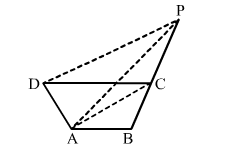# In the adjoining figure, ABCD is a quadrilateral. A line through D, parallel to AC, meets BC produced in P.

Question:

In the adjoining figure, ABCD is a quadrilateral. A line through D, parallel to AC, meets BC produced in P. Prove that ar(ABP) = ar(quad. ABCD).Solution:

We have:
​ar(quad. ABCD) = ar(∆ACD) + ar(∆ABC)
ar(∆ABP) = ar(∆ACP)​​ + ar(∆ABC)

ACD and ∆ACP are on the same base and between the same parallels AC and DP.
∴ ar(∆ACD) = ar(∆ ACP)​
By adding ar(∆ABC) on both sides, we get:
ar(∆ACDar(∆ABC) = ar(∆ACP)​​ + ar(∆ABC)
⇒​ ar (quad. ABCD) = ar(∆ABP)
Hence, proved.